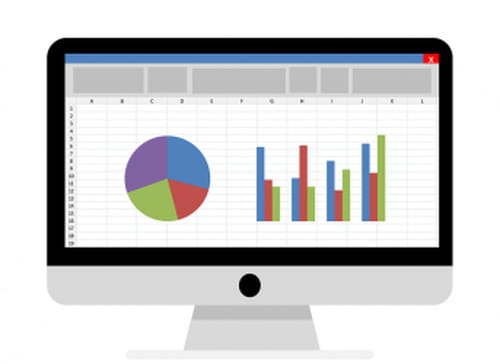0
Thanks

A few words of thanks would be greatly appreciated.

# Excel - Find occurences of same value in a column

<ital>

If you would like to find out how many occurrences of the same number occur in a column in Excel, keep reading.<ital>## Example

1111155555555666666666666 (vertical column, although not depicted here)

`1's=50 5's=8 6's=12 `

We have merged multiple columns into a single column totalling 5000 and we have sorted them numerically, but there are just too many to manually count.

So, if the numbers are different cells in a column and if the total no. of rows is 5000. We can use this formula:

`=countif(a1:a5000,"1") `

In case of a whole string or number in one cell we should use this (supposing the cell is E1):

`=LEN(E1)-LEN(SUBSTITUTE(E1,"1","")) `